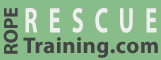# Fall Factors

When a rope catches a falling load, the forces generated are based on the weight of the load, the distance that the load falls, and the length of the rope between the load and the anchor. The relationship between the length of the rope and the distance the load falls is called the fall factor. The fall factor is calculated by dividing the distance that the load falls by the length of the rope.For example, if a load falls 4 feet when secured by 8 feet of rope, the fall factor is 0.5.## It's the Factor

It is not intuitive, but falling 20 feet on 40 feet of rope creates similar forces, and the same fall factor, as falling 4 feet on 8 feet of rope or falling 1 foot on 2 feet of rope—a 0.5 fall factor.Of course, the likelihood that the rescuer will be injured during the fall by hitting other objects is much greater during a 20-foot fall than in a 4-foot fall, but the impact forces will be similar.

The other non-inconsequential risk is hitting the ground. Falling when you are 10 feet above the ground while attached to 11 feet of rope is bad.

## The Danger Zone

The fall factor math works because it compares the distance the load falls with the amount of rope that can absorb the energy by stretching. Even if the rescuer only takes a small fall while attached to a very short rope, the forces can be very high. For example, falling a meter on a meter of rope, a fall factor of 1.0, generates tremendous force.

The greatest risk of a high fall factor accident is when there is slack in the system and you are close to the anchor. For rescuers, this is when we are approaching the edge. And if you increase the load by combining a rescuer and patient, the forces can be gear-snapping.

## Maximum Fall Factor

Generally speaking, the maximum fall factor for rescuers and climbers is 2.0. This happens when the rescuer falls twice the length of his rope. Falling twice the length of the rope generates a 2.0 fall factor regardless of whether the climber falls 2 feet on 1 foot of rope or falls 20 feet on 10 feet of rope. A 2.0 fall factor is most likely to occur when a rescuer is tied into an anchor with a relatively short piece of material, climbs that distance above the anchor, and falls. A 2.0 fall factor is almost certain to cause injuries unless there is something in the system to absorb the impact.

It is possible, although unusual, to have a fall factor that is greater than 2.0. This can happen if the rope attachment can travel, as in this illustration which shows a 4.0 fall factor.

## How Much is Too Much?

OSHA specifies a maximum fall distance of 6 feet regardless of the fall factor. Although they don't specify the maximum fall factor, they do specify that the maximum force must not exceed 4 kN (900 pounds) when using a body belt and 8 kN (1800 pounds) with a body harness.

The following data were generated using this calculator and assume that the rope has 10% elongation. (Note that this calculator makes assumptions that we haven't validated.)

• A 165 pound (75 kg) rescuer who experiences a 0.25 fall factor (e.g., falling 2 feet on 8 feet of rope) will generate ~4 kN of force (the maximum for a body belt).
• A 165 pound (75 kg) rescuer who experiences a 2.0 fall factor (e.g., falling 6 feet on 3 feet of rope) would generate ~6 kN.
• A 225 pound (100 kg) rescuer who experiences a 0.25 fall factor (e.g., falling 2 feet on 8 feet of rope) will generate ~4 kN of force (the maximum for a body belt).
• A 225 pound (100 kg) rescuer who experiences a 1.5 fall factor (e.g., falling 6 feet on 4 feet of rope) would generate ~8 kN.

## The Small Print

The actual forces that are generated by a fall are a function of the load, the fall factor, and how much energy is absorbed by the ropes, knots, harness, and the rescuer's body. For example, a 0.5 fall factor on dynamic climbing rope will generate much less force than the same fall on a low-stretch rescue rope.

The fall factor concept, that the force remains constant if the fall factor remains constant, is sound theory: it isn't the length of the fall, but rather it is the length of the fall compared to the amount of rope that can absorb the energy. However, during testing the actual measured forces do increase with longer falls when using low-stretch (i.e., "static") rope even if the fall factor remains constant. This increase in force is relatively small at a fall factor of 0.25, but at higher fall factors the increase in force is significant.

That said, fall factors remain a very important concept. Rescuers must understand that higher fall factors dramatically increase impact forces and they must diligently seek to minimize potential fall factors.

Try It.
Risk Free.